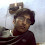## Monday, May 19, 2008

### Geometry Problem 99See complete Problem 99
Circle Area, General Extension to Pythagoras' Theorem. Level: High School, SAT Prep, College geometry

#### 1 comment:

1.If we take the area of semicricle with diameter AO as x and semicircle woth diameter DC as y, then by pythagoras theorem on the trianle ABC, we can say that
S+x=S1+S2+y

Now using the pythagoras theorem in right angled triangle DOC,
We can say that
y=x+S3

putting this value of y in above equation we can prove that

S=S1+S2+S3